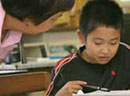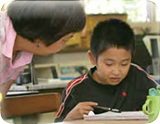# Measure Up

### Strong STEM Focus ContinuesCounting discrete objects is a common basis for introducing children to mathematical ideas. A different approach in teaching mathematics to first graders is to have students begin with generalized notions without using number. R esearch in the Measure Up program introduces algebraic concepts as early as the first grade through the exploration of fundamental mathematical relationships, such as equality and inequality, before students are introduced to the concept of number.